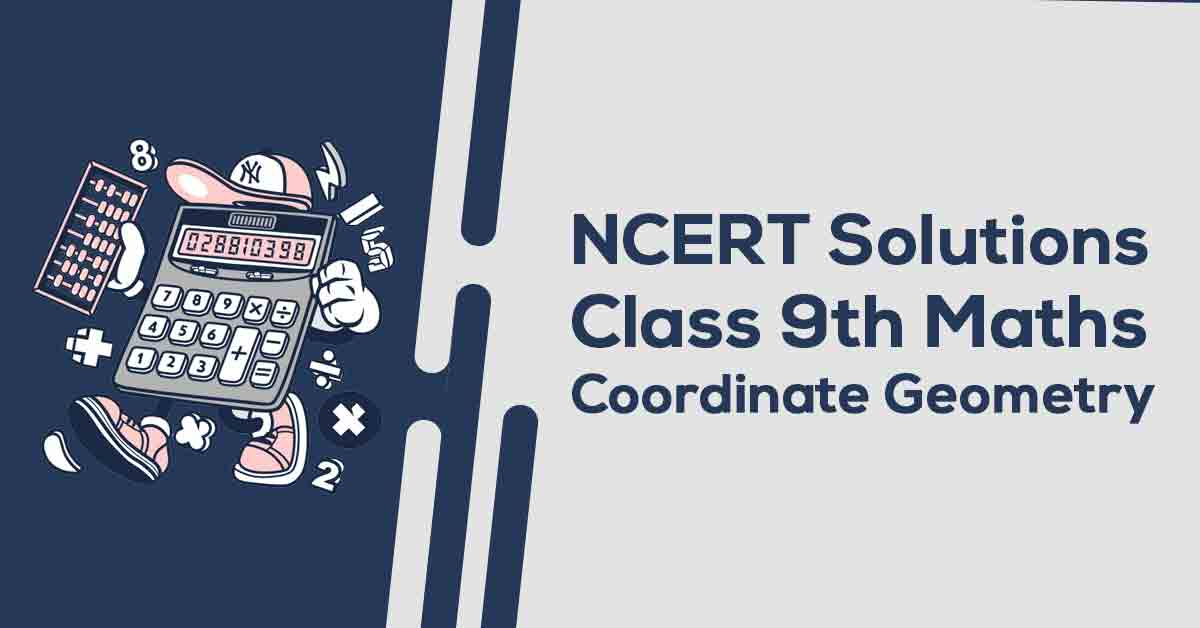Class 9th Math Coordinate Geometry NCERT Solution CBSE 2023

# NCERT Solutions For Class 9 Maths Chapter 3

## NCERT Solutions For Class 9 Maths Chapter 3 Coordinate Geometry - PDF DownloadStudents can take help from NCERT Solutions for class 9 chapter 3 Coordinate Geometry to study for their examinations. In this Chapter, we will learn to locate the position of an object or a point in a plane, we require two perpendicular lines. One of them is horizontal, and the other is vertical. The plane is called the Cartesian, or coordinate plane and the lines are called the coordinate axes. The horizontal line is called the x-axis, and the vertical line is called the y-axis.

These CBSE NCERT Solutions for Class 9 Maths Chapter 3 Coordinate Geometry will help you to practice all the topics in the chapter with ease and score maximum marks in the examination.

What id Coordinate Geometry?

Coordinate Geometry is defined as the geometry in which we can use graphs and coordinates to find measurements and other useful information about geometric figures.